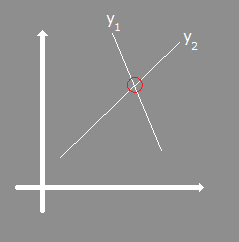What is line intersection?

A line intersection is present if two lines have one point in common.

What will this site do for you?

This site will calculate the line intersection point of two lines described by two linear functions.

There are 3 possible outcomes:
• No result, as the lines go parallel
• Infinite results, as the lines are described with the same function
• One result, if both lines have exactly one point in common

You have to enter two functions that describe the two lines that should be checked if they have an intersection point. The function follows the pattern y = ax + b where ...
• ... y1, y2 are the dependent variables
• ... x is the independent variable
• ... a1, a2 are the slope of the line
• ... b1, b2 are the intersection point with the y-axis
Lines Intersection

Calculates the intersection point of two lines.y1 = · x + y2 = · x +